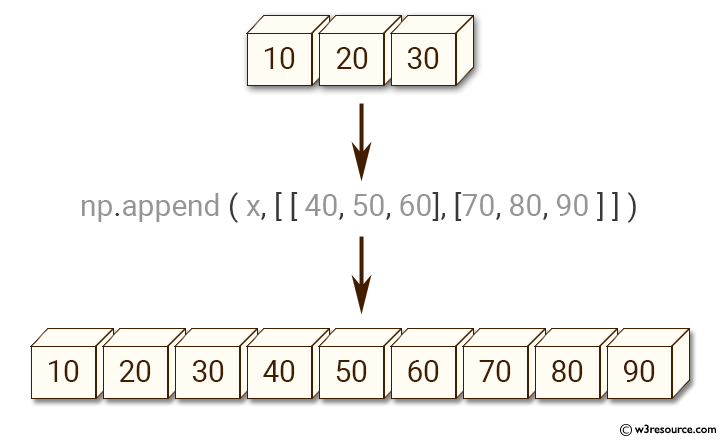﻿ NumPy: Append values to the end of an array - w3resource# NumPy: Append values to the end of an array

## NumPy: Array Object Exercise-12 with Solution

Write a NumPy program to append values to the end of an array.Sample Solution:-

Python Code:

``````import numpy as np
x = [10, 20, 30]
print("Original array:")
print(x)
x = np.append(x, [[40, 50, 60], [70, 80, 90]])
print("After append values to the end of the array:")
print(x)
```
```

Sample Output:

```Original array:
[10, 20, 30]
After append values to the end of the array:
[10 20 30 40 50 60 70 80 90]
```

Python Code Editor:

Have another way to solve this solution? Contribute your code (and comments) through Disqus.

What is the difficulty level of this exercise?

Test your Python skills with w3resource's quiz

﻿

## Python: Tips of the Day

Set comprehension:

```>>> m = {x ** 2 for x in range(5)}
>>> m
{0, 1, 4, 9, 16}
```